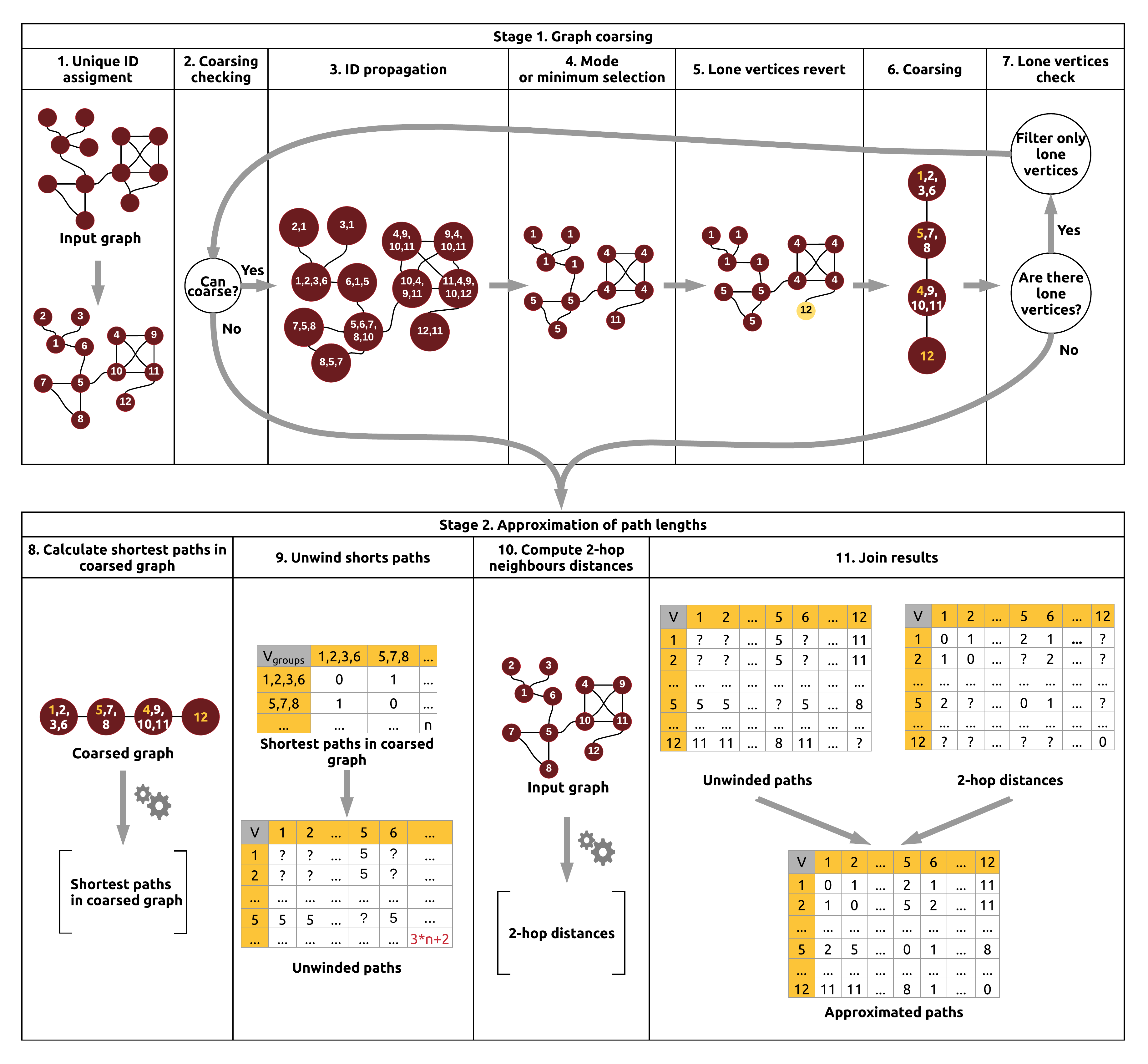# Shortest paths approximation¶

In order to limit computation time of shortest paths for large graphs, library gives ability to approximate them. Approximation can be divided into four main phases:

1. Graph coarsening
2. Paths calculation in coarsed graph
3. 2-hop neighborhood distances calculation
4. Paths approximation

Approximation gives worst-case result of 3*p+2 where p is real path. Result is not awesome in terms of beeing exact, but it keeps rankings of vertices and can be used for measures approximation (Closeness) or in tasks where order of vertices is important, not exact distance.

## Alghotim block scheme¶## Examples¶

Alghoritm API lets to compute paths :

• For single vertex:

import ml.sparkling.graph.operators.algorithms.aproximation.ApproximatedShortestPathsAlgorithm
import org.apache.spark.SparkContext
import org.apache.spark.graphx.{Graph, VertexRDD}

implicit ctx:SparkContext=???
// initialize your SparkContext as implicit value
val graph = ???
val sourceVertexId=1
val graphWithPaths=ApproximatedShortestPathsAlgorithm.computeSingleShortestPathsLengths(graph,sourceVertexId)
val paths : VertexRDD[Iterable[(VertexId, JDouble)]  =  graphWithPaths.vertices

• For whole graph:

import ml.sparkling.graph.operators.algorithms.aproximation.ApproximatedShortestPathsAlgorithm
import org.apache.spark.SparkContext
import org.apache.spark.graphx.{Graph, VertexRDD}

implicit ctx:SparkContext=???
// initialize your SparkContext as implicit value
val graph = ???
val graphWithPaths =  ApproximatedShortestPathsAlgorithm.computeShortestPaths(graph)
val paths : VertexRDD[Iterable[(VertexId, JDouble)]  =  graphWithPaths.vertices

• using iterative approach:

import ml.sparkling.graph.operators.algorithms.aproximation.ApproximatedShortestPathsAlgorithm
import org.apache.spark.SparkContext
import org.apache.spark.graphx.{Graph, VertexRDD}

implicit ctx:SparkContext=???
// initialize your SparkContext as implicit value
val graph = ???